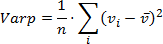.NET Framework 4.5.2+

# PivotDataConsolidationFunction Enum

Lists aggregation functions to apply to data fields in a PivotTable report.

## Declaration

``public enum PivotDataConsolidationFunction``

## Members

Name Description
`Average`

The average of the values.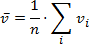`Count`

The number of nonempty values.

`CountNumbers`

The number of numeric values.

`Max`

The largest value.

`Min`

The smallest value.

`Product`

The product of the values.

`StdDev`

An estimate of the standard deviation of a population, where the sample is a subset of the entire population.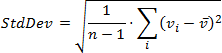`StdDevp`

The standard deviation of a population, where the population is all of the data to be summarized.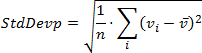`Sum`

The sum of the values.

`Var`

An estimate of the variance of a population, where the sample is a subset of the entire population.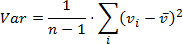`Varp`

The variance of a population, where the population is all of the data to be summarized.